Courses

# Chapter Notes - Perimeter and Area Class 7 Notes | EduRev

## Class 7 : Chapter Notes - Perimeter and Area Class 7 Notes | EduRev

The document Chapter Notes - Perimeter and Area Class 7 Notes | EduRev is a part of the Class 7 Course Mathematics (Maths) Class 7.
All you need of Class 7 at this link: Class 7

11. Perimeter and Area

Plane Figures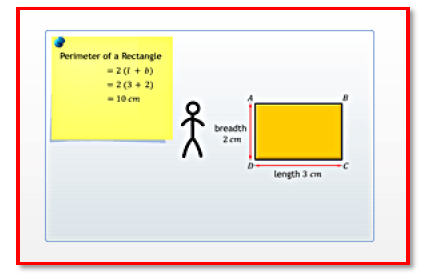The area of the parallelogram is given by base x height.

Triangle: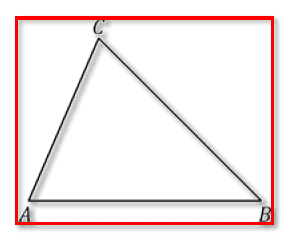A triangle is a polygon with three vertices, and three sides or edges that are line segments. A triangle with vertices A, B, and C is denoted as ABC

The perimeter of a triangle is the sum of the lengths of its sides. If the three sides are a, b, and c, then perimeter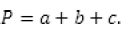Page 43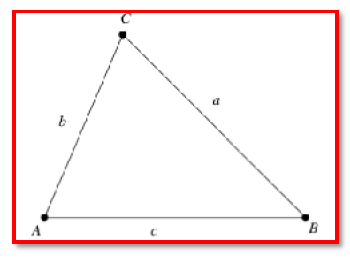The area of a triangle is the space enclosed by its three sides. It is given by the formula,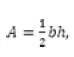where b is the base and h is the altitude.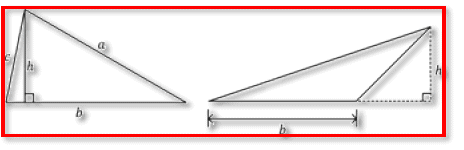A simple closed figure bounded by four line segments is called a quadrilateral.

Rectangle
Square
Parallelogram
Rhombus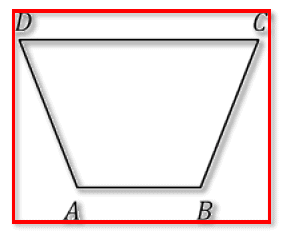A rectangle is a quadrilateral with opposite sides equal, and each angle of measure 90o.

Page 44

The perimeter of a rectangle is twice the sum of the lengths of its adjacent sides.
In the figure, the perimeter of rectangle ABCD = 2(AB + BC).

The area of a rectangle is the product of its length and breadth.
In the figure, the area of rectangle ABCD = AB x BC.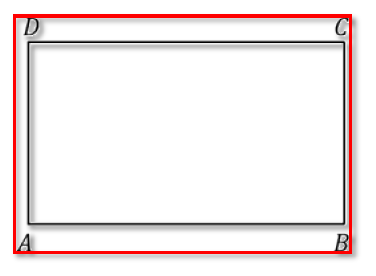A square is a quadrilateral with four equal sides, and each angle of measure 90o.

The perimeter of a square with side s units is 4s.

In the figure, the perimeter of square ABCD = 4AB or 4BC or 4CD or 4DA.

The area of a square with side s is s2

In the figure, the perimeter of square ABCD = AB2 or BC2 or CD2 or DA2.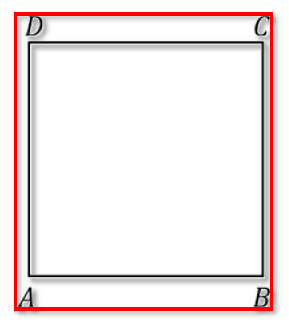Page 45

A quadrilateral in which both the pairs of opposite sides are parallel is called a parallelogram.

The perimeter of a parallelogram is twice the sum of the lengths of the adjacent sides.

In the figure, the perimeter of parallelogram ABCD = 2(AB + BC)

The area of a parallelogram is the product of its base and perpendicular height or altitude.

Any side of a parallelogram can be taken as the base. The perpendicular dropped on that side from the opposite vertex is known as the height (altitude).

In the figure, the area of parallelogram ABCD = AB x DE or AD x BF.A parallelogram in which the adjacent sides are equal is called a rhombus.

The perimeter and area of a rhombus can be calculated using the same formulae as that for a parallelogram.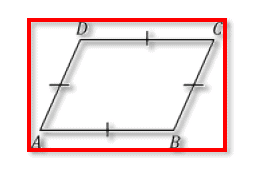Page 46

Circles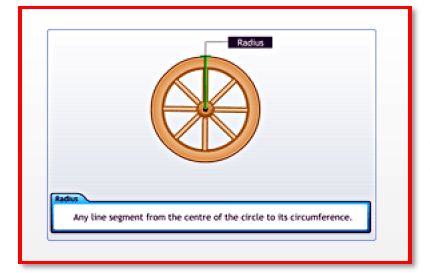A circle is defined as a collection of points on a plane that are at an equal distance...

Circle:

A circle is defined as a collection of points on a plane that are at an equal distance from a fixed point on the plane. The fixed point is called the centre of the circle.

Circumference:

The distance around a circular region is known as its circumference.

Diameter:

Any straight line segment that passes through the centre of a circle and whose end points are on the circle is called its diameter.

Any line segment from the centre of the circle to its circumference.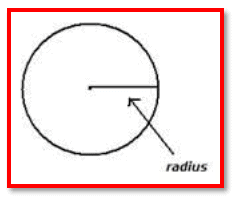Page 47

Circumference of a circle =  2πr, where r is the radius of the circle or , where πd is the diameter of the circle.

Π is an irrational number, whose value is approximately equal to .

Circumference = Diameter x 3.14

Diameter(d) is equal to twice radius(r). d=2r

Circles with the same centre but different radii are called concentric circles.

Circle:

The area of a circle is the region enclosed in the circle.

The area of a circle can be calculated by using the formula: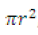if radius r is given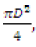if diameter D is given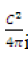if circumference C is given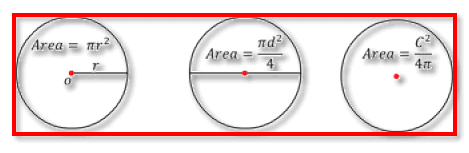Offer running on EduRev: Apply code STAYHOME200 to get INR 200 off on our premium plan EduRev Infinity!

## Mathematics (Maths) Class 7

210 videos|109 docs|45 tests

,

,

,

,

,

,

,

,

,

,

,

,

,

,

,

,

,

,

,

,

,

;